# Simple introduction to Regression

Predictive analytics deals with the analysis of the historical and current data to predict the future or unknown. Some of the instances of predictive analytics are prediction of if a customer might might end their relationship with a company: Customer churn prediction, prediction of demand of a product using information like prior history of the product which includes information like number of products sold, regions of demand, seasonality, competitors’ new products in the market, etc. — Sales forecasting. Regression is one of the techniques used in predictive analytics.

# Regression

Regression is a statistical technique and a supervised algorithm which models the relationship among variables. What are these variables? there are 2 types of variables:

1. Independent variable: Usually called as ‘x’

Regression models the relationship between the one/more dependent variables and one/more independent variables. It tries to fit a best fit line for the training data, the aim is to have low prediction error.

Some of the instances where we can use regression are, can I predict how happy (as real number) a kid is on his/her birthday? Based on the number of gifts, size of the gifts, likability of the gifts, etc. If I know this, then I can plan better for the next year birthday. Another example, can we predict how satisfied employees are? Based on the type of job, type of projects, work-life balance, etc.

Precisely, dependent variable (y) from the above examples are happiness, satisfaction and independent variables (x’s) are the number of gifts, size of the gifts, likability of the gifts, the type of job, type of projects, work-life balance.

# Types of regression.

There are many types of regression models

1. Linear Regression

Let’s start with Linear Regression

# Linear Regression

A linear regression models the relationship between a dependent variable (y) and an independent variables (x) by fitting a best fit regression line. Under the hood, it is a weighted sum of x along with a bias/ intercept.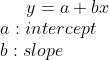Linear Regression equation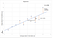Fig 1: Linear Regression with one independent variable

Fig 1 shows data points, intercept, best fit line and the error between the actual and predicted values.

One of the error metrics for regression is Root Mean Square Errors(RMSE). RMSE is the square root of averaged errors, where error is the difference between the predicted and actual values.

Other error metrics for regression are Mean Absolute Error, Mean Squared Error,R² or Coefficient of Determination and Adjusted R².

But how to get the best fit line and reduce the RMSE? Using Normal equation, optimization algorithms like gradient descent (which will be discussed in upcoming blogs)

# Polynomial Regression

What happens when a non-linear relationship exists between X and Y? If linear regression is used then the prediction error will be high. In such cases, we can use polynomial regression which uses polynomial features along with the existing features shown in fig 3.

The degree of the above equation is 2.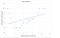Fig 2: High error exists when linear regression is used with non linear data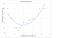Fig 3: Polynomial regression

With polynomial regression, overfitting can be a consequence of increasing the degree of the equation to fit the data perfectly.

A model is said to be overfitted when the model is tailored to the training data to a high degree and fails to generalize on the new unseen data.

One of the disadvantages, of polynomial regressions is it is sensitive to outliers.

Outliers in simple term means observations which are not similar to normal observations. Outliers can be considered as one-off events, error in recording observations, etc.

How to avoid overfitting? regularization is a technique to prevent overfitting.

# Ridge Regression

Ridge Regression is a modified form of the linear regression, it is a regularized technique. In this type, the cost function is modified by adding square of the magnitude of the coefficients. What happens with that? The model tries to fit the data with the constraint of keeping the coefficients as small as possible and the cost function low. This reduces the complexity of the model.

α = 0, means no regularization (similar to linear regression)

α = Very large value, means all the coefficients will be close to zero.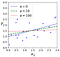Fig 4: Ridge regression with various values of alpha (Géron, A. 2019)

From the above figure, we can see that we obtain different regression lines with different values of alpha.

# Lasso Regression (least absolute shrinkage and selection operator)

Lasso regression is another regularized Linear Regression model. The cost function is modified by adding the magnitude of coefficients . There are 2 important points to be considered

1. Avoids overfitting.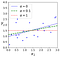Fig 5: Lasso Regression (Géron, A. 2019)

# Elastic Net

Elastic Net is a mix of both Ridge and Lasso regression. By controlling the value of r in the regularization term, we can decide the contribution of each type of regression.

r=0: Elastic Net is equal to Ridge regression

r =1: Elastic Net is equal to Lasso regression

Thank you for reading.

## References

1. Géron, A. (2019). Ridge Regression [Image]. In Hands-on Machine Learning with Scikit-Learn, Keras, and TensorFlow (p. 138).

I am a data scientist. I write blogs related to machine learning.

## More from Nanda

I am a data scientist. I write blogs related to machine learning.